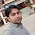## OBJECTIVE QUESTIONS FROM BASIC ELECTRICAL ENGINEERING:

 The power plant having highest efficiency is
A.  Hydro
B.  Thermal
C.  Nuclear
D.  Diesel

 As the spacing between plates in a capacitor is made smaller
A.  The capacitance increases
B.  The capacitance decreases
C.  The capacitance does not change
D.  The resistance increases

 The relationship between frequency and time period of ac waveform is
A.  f = T
B.  f = 1/ T2
C.  f = 1/T
D.  f = T2
 The five 0.050uF capacitors are connected in parallel. The equivalent capacitance is
A.  0.010 uf
B.  0.25 uf
C.  0.50uf
D.  0.025uf

 The current gain of a bipolar transistor drops at high frequencies because of
A. Transistor capacitance
B. High current effects in the base
C. Parasitic inductive elements
D. The early effect

 If two perfect sine waves have the same frequency and the same amplitude, but are in opposite phase, the composite wave
A.  Has twice the amplitude of either input wave alone
B.  Has half the amplitude of either input wave alone
C.  Is complex, but has the same frequency as the originals.
D.  Has zero amplitude ( that is, it does not exist) because the two input waves cancel each other out.

 Three capacitors are connected in series having capacitance of 8uF, 32uF, 16uF, the total capacitance is
A. 32/7 uF
B. 7.32 uF
C. 56 uF
D. 32 uF
 Which one of the following does not affect the power output available from a particular ac generator?
A.  The strength of the magnet
B.  The number of turns in the coil
C.  The type of natural energy source used
D.  The speed of rotation of the coil or magnet

 Which of the following are active components
(a) Resistor and inductor
(b) Diode, BJT, FET
(c) Opamp, BJT, thermionic triode
(d) Capacitor, Inductor

 The energy required to charge a 10 uF capacitor to 100V is
(a) 0.10 J
(b) 0.05 J
(c) 5 X 10-9 J
(d) 10 X 10-9 J

 The current in a inductor changes from 0 to 200mA in 4ms and induces a voltage of 100mV. The value of inductor is
(a) 2mH
(b) 0.5mH
(c) 8mH
(d) 4mH

 A battery has a short-circuit current of 30A and an open circuit voltage of 24V. If the battery is connected to an electric bulb of resistance 2 ?, the power dissipated by the bulb is
(a) 80W
(b) 1800W
(c) 112.5W
(d) 228W

 Which of the following is ferromagnetic material?
(a)  Copper
(b)  Palladium
(c)  Silver
(d)  Cobalt

 A complex wave form made up frequency components 1 Hz, 3 Hz, 5 Hz, 7 Hz and 9 Hz. Its fundamental frequency is
(a)  9 Hz
(b)  12.5 Hz
(c)  1 Hz
(d)  Indeterminate

 If a 175V dc source were connected in series with the utility mains from a standard wall outlet, the result would be
(a)  Smooth dc at a constant voltage
(b)  Pure ac with equal peak voltages
(c)  Ac with one peak voltage greater than the other
(d)  Fluctuating dc

10:43 AM

#### 3 comments:

1.can anyone explain Q.15 answer ????

2.Please Explain Q.10; Q 12; Q.14

3.question 1 ans is wrong -right answar is hydro